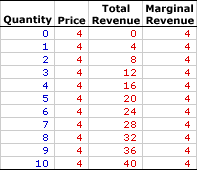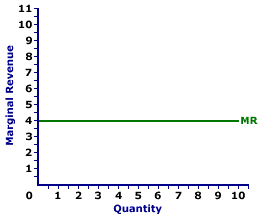Wednesday  June 29, 2022
 AmosWEB means Economics with a Touch of Whimsy!AFC: The abbreviation for average fixed cost, which is fixed cost per unit of output, found by dividing total fixed cost by the quantity of output. Average fixed cost is one of three related cost averages. The other two are average variable cost and avarage total cost. Average fixed cost decreases with larger quantities of output. Because fixed cost is FIXED and does not change with the quantity of output, a given cost is spread more thinly per unit as quantity increases. A thousand dollars of fixed cost averages out to \$10 per unit if only 100 units are produced. But if 10,000 units are produced, then the average shrinks to a mere 10 cents per unit.MARGINAL REVENUE, PERFECT COMPETITION:

The change in total revenue resulting from a change in the quantity of output sold. Marginal revenue indicates how much extra revenue a perfectly competitive firm receives for selling an extra unit of output. It is found by dividing the change in total revenue by the change in the quantity of output. Marginal revenue is the slope of the total revenue curve and is one of two revenue concepts derived from total revenue. The other is average revenue. To maximize profit, a perfectly competitive firm equates marginal revenue and marginal cost.
Marginal revenue is the extra revenue generated when a perfectly competitive firm sells one more unit of output. It plays a key role in the profit-maximizing decision of a perfectly competitive firm relative to marginal cost. A perfectly competitive firm maximizes profit by equating marginal revenue, the extra revenue generated from production, with marginal cost, the extra cost of production. If these two marginals are not equal, then profit can be increased by producing more or less output.

The relation between marginal revenue and the quantity of output produced depends on market structure. For a perfectly competitive firm, marginal revenue is equal to price and average revenue, all three of which are constant. For a monopoly, monopolistically competitive, or oligopoly firm, marginal revenue is less than average revenue and price, all three of which decrease with larger quantities of output. The constant or decreasing nature of marginal revenue is a prime indication of the market control of a firm.

Marginal revenue can be represented in a table or as a curve. For a perfectly competitive firm, the marginal revenue curve is a horizontal, or perfectly elastic, line. For a monopoly, oligopoly, or monopolistically competitive firm, the marginal revenue curve is negatively sloped.

The marginal revenue received by a firm is the change in total revenue divided by the change in quantity, often expressed as this simple equation:

 marginal revenue = change in total revenuechange quantity

Perfect competition is a market structure with a large number of small firms, each selling identical goods. Perfectly competitive firms have perfect knowledge and perfect mobility into and out of the market. These conditions mean perfectly competitive firms are price takers, they have no market control and receive the going market price for all output sold.

### Some Numbers

Marginal Revenue,
Perfect CompetitionThe table to the right summarizes the marginal revenue received by a hypothetical firm, Phil the zucchini grower, for selling zucchinis in a perfectly competitive zucchini market. The first column is the quantity of zucchinis sold, ranging from 0 to 10 pounds. The second column is then the price Phil receives for selling his zucchinis, which is \$4 per pound. The third column is the total revenue Phil receives for producing and selling alternative quantities of zucchinis.

Marginal revenue in the forth column is found by dividing the change in total revenue (from the third column) by the change in quantity (from the first column). For example, when Phil increases production and sales from 4 pounds of zucchinis to 5 pounds, his total revenue increases from \$16 to \$20, an increase of \$4. As such, the marginal revenue of producing the fifth pound of zucchinis is \$4 (= \$4/1). Each value in the fourth column is calculated in the same way.

The obvious point is that marginal revenue is equal to \$4 for every extra pound of zucchinis sold. This results because price is constant at \$4 for every pound of zucchinis sold. For every extra pound of zucchinis sold by Phil, he receives an extra \$4, which is the going market price. Marginal revenue is equal to price for a perfectly competitive firm.

### The Curve

Marginal Revenue Curve,
Perfect CompetitionMarginal revenue is commonly represented by a marginal revenue curve, such as the one labeled MR and displayed in the exhibit to the right. This particular marginal revenue curve is also that for zucchini sales by Phil the zucchini grower, a presumed perfectly competitive firm.

The vertical axis measures marginal revenue and the horizontal axis measures the quantity of output (pounds of zucchinis). Although quantity on this particular graph stops at 10 pounds of zucchinis, the nature of perfect competition indicates it could easily go higher.

This curve indicates that if Phil sells the first pound of zucchinis (an increase in production from 0 to 1), then his extra revenue is \$4. However, if he sells his tenth pound (an increase in production from 9 to 10), then he also receives \$4 of extra revenue. Should he sell his hundredth pound (an increase in production from 99 to 100), then he moves well beyond the graph, but his marginal revenue remains at \$4.

Because Phil is a perfectly competitive firm, his marginal revenue curve is also his demand curve and his average revenue curve. All three curves coincide for perfect competition.

 <= MARGINAL REVENUE, MONOPOLY MARGINAL REVENUE PRODUCT =>Recommended Citation:

MARGINAL REVENUE, PERFECT COMPETITION, AmosWEB Encyclonomic WEB*pedia, http://www.AmosWEB.com, AmosWEB LLC, 2000-2022. [Accessed: June 29, 2022].

Check Out These Related Terms...

Or For A Little Background...

And For Further Study...

Related Websites (Will Open in New Window)...
Search Again?ORANGE REBELOON[What's This?] Today, you are likely to spend a great deal of time at a dollar discount store seeking to buy either a T-shirt commemorating the first day of winter or software that won't crash your computer. Be on the lookout for fairy dust that tastes like salt.Your Complete ScopeThe standard "debt" notation I.O.U. does not mean "I owe you," but actually stands for "I owe unto...""Concentration is the secret of strength in politics, in war, in trade, in short in all management of human affairs. "-- Ralph Waldo Emerson, philosopher, poetMCAMonetary Control Act of 1980A PEDestrian's Guide Xtra CreditTell us what you think about AmosWEB. Like what you see? Have suggestions for improvements? Let us know. Click the User Feedback link.| | | | | | | | | | |
| | | |

Thanks for visiting AmosWEB